×

Search anything:

# Heap Sort

#### Sorting Algorithms Algorithms heap sort Problems on Binary TreeGet this book -> Problems on Array: For Interviews and Competitive Programming

Reading time: 25 minutes | Coding time: 10 minutes

Heapsort is an efficient in-place comparison based sorting algorithm with O(N log N) time complexity and uses a data structure to achieve it. It uses a complete Binary Heap data structure to sort the elements depending on whether the heap is Min Heap (ascending) or Max heap (descending). A binary heap is a complete binary tree, that is, all the levels of the tree are completely filled except, possibly the last level. And the insertion of elements is done from the left and goes to right.

### Algorithm

Consider that we are using Max heap as the underlying structure for heapsort.

Max heap is a binary heap such as the root node is larger than all nodes that are a part of its left and right sub trees which are in turn max heap. An instant insight is that the root node of a max heap is the maximum element of the set of elements

Hence, the first step is to create a Max heap from the given set of N elements. This step takes O(N) time complexity

Second step is to remove the root element which is the largest element and replace it with the bottom most node which is a left. Hence, the set of elements has now N-1 elements and the tree is not a max heap. To make it a Max heap again, we need to check each node and swap the parent and child nodes accordingly. This step takes O(log N) time complexity.

The above step is repeated N-1 times till we are left with only one element.

Hence, the overall complexity is O(N log N) and it uses are fundamental property of binary heaps to sort a dataset.

### Complexity

• Worst case time complexity: `Θ(nlogn) `
• Average case time complexity: `Θ(nlogn)`
• Best case time complexity: `Θ(nlogn)`
• Space complexity: `Θ(1)`

### Pseudocode

``````
procedure heapsort(array, n):
// Step 1: creating the initial Max heap
for i = n/2 - 1 to 0 do:
heapify(array, n, i)
// Step 2: Removing one element and maintaining the heap property
for i = n-1 to 0 do:
swap(array, array[i])
heapify(array, i, 0)

procedure heapify(array, n, i)
// initialize largest as root and get left and right nodes
int largest = i;
int left_node = 2*i + 1;
int right_node = 2*i + 2;
// Check if left node exists and is larger than root. If yes, change it
if(left_node < n && arr[left_node] > arr[largest])
largest = left_node;
// Check if right node exists and is larger than root. If yes, change it
if(right_node < n && arr[right_node] > arr[largest])
largest = right_node;
// change root, if needed
if(largest != i)
swap(arr[i], arr[largest]);
heapify(arr, n, largest);
``````

### Example

Dataset: 4 10 3 5 1

We create a heap which we covert to a Max Heap in O(N) time complexity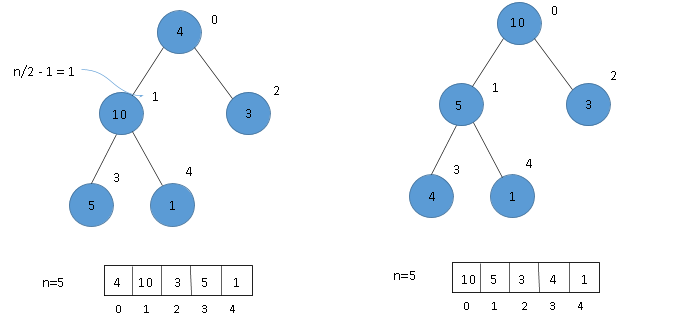(1) Building a Heap tree. (2) Creating a Max heap in which child nodes are less than root node.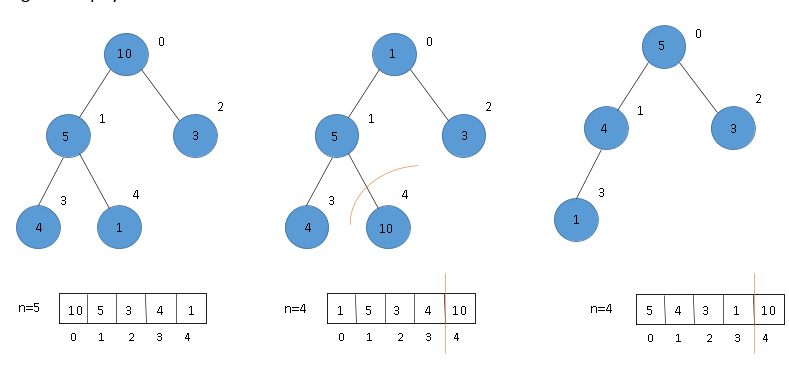(2) Swap 1st and last nodes (10 and 1) and build heap again. (3) Exclude last node and heapify again.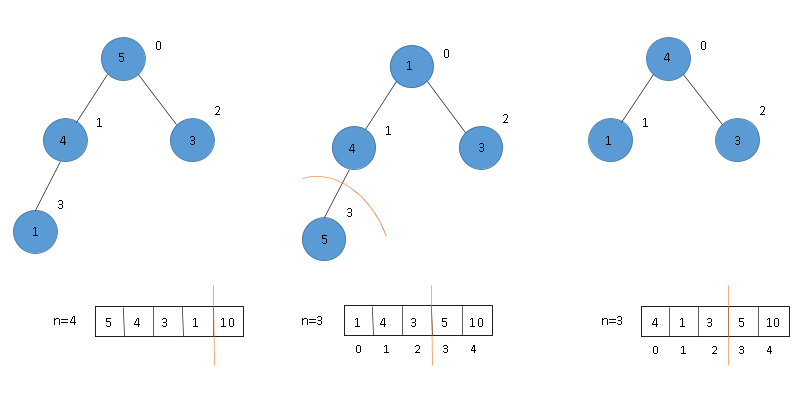(2) Swap 1st and last node (5 and 1) and build heap. (3) Exclude last node and heapify.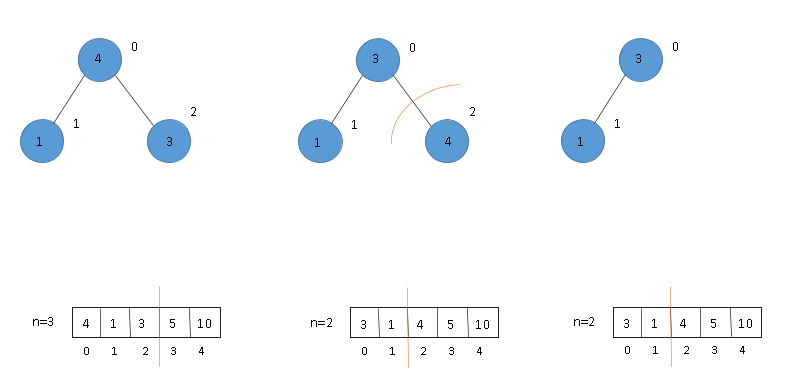(2) Swap 1st and last node (4 and 3) and build heap. (3) Exclude last node and heapify.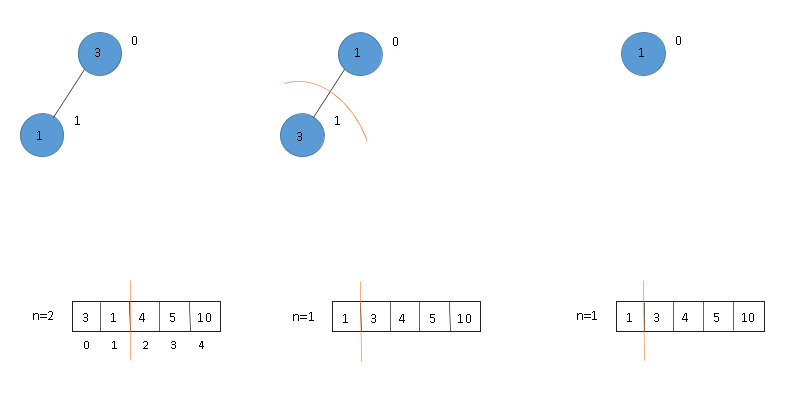(2) Swap 1st and last node (3 and 1) and build heap. (3) Exclude last node and heapify. Final array is sorted.

• C

### C

``````
#include <stdio.h>
void heapsort(int arr[], int n);
void heapify(int arr[], int n, int i);
void swap(int* a, int* b);
int main()
{
int n, i;
printf("Enter number of elements to input: ");
scanf("%d", &n);
int arr[n];
printf("Enter the elements\n");
for(i = 0; i < n; i++)
scanf("%d", &arr[i]);
heapsort(arr, n);
printf("Elements after sorting\n");
for(i = 0; i < n; i++)
printf("%d ", arr[i]);
}
void heapsort(int arr[], int n)
{
int i;
// Build a max heap
for(i = n/2 - 1; i >= 0; i--)
heapify(arr, n, i);
// One by one extract elements
for(i = n-1; i >= 0; i--)
{
swap(&arr, &arr[i]);
heapify(arr, i, 0);
}
}
void heapify(int arr[], int n, int i)
{
// initialize largest as root and get left and right nodes
int largest = i;
int left_node = 2*i + 1;
int right_node = 2*i + 2;
// Check if left node exists and is larger than root. If yes, change it
if(left_node < n && arr[left_node] > arr[largest])
largest = left_node;
// Check if right node exists and is larger than root. If yes, change it
if(right_node < n && arr[right_node] > arr[largest])
largest = right_node;
// change root, if needed
if(largest != i)
{
swap(&arr[i], &arr[largest]);
heapify(arr, n, largest);
}
}
void swap(int* a, int* b)
{
int temp = *a;
*a = *b;
*b = temp;
}
``````

### Applications

1. Finding extremas - Heap sort can easily be used find the maxima and minimum in a given sequence of numbers.
2. Job Scheduling - In Linux OS, heapsort is widely used for job scheduling of processes due to it's O(nlogn) time complexity and O(1) space complexity.
3. Graph Algorithms - It can used in the implementation of priority queue for Djikstra's algorithm, Prim's algorithm and Huffmann encoding as well.

1. Heap sort is not a stable sort and requires constant space for sorting.
2. Memory management is quite complex due to heap data structure.

### Questions

###### Question 1: In a binary max heap containing n numbers, the smallest element can be found with what time complexity?
O(n)
O(logn)
O(loglogn)
O(1)
In a max heap the smallest element will be placed at the far-right corner of the heap array. Hence it will take n searches to find the smallest element. (Note - Max heap is not sorted generally.)
###### Question 2: Heap sort is an in-place algorithm. True or False?
True
False
Yes, Heap sort is an in-place algorithm because the input array is itself transformed into a max heap and then heapified without using any other array or data structure.